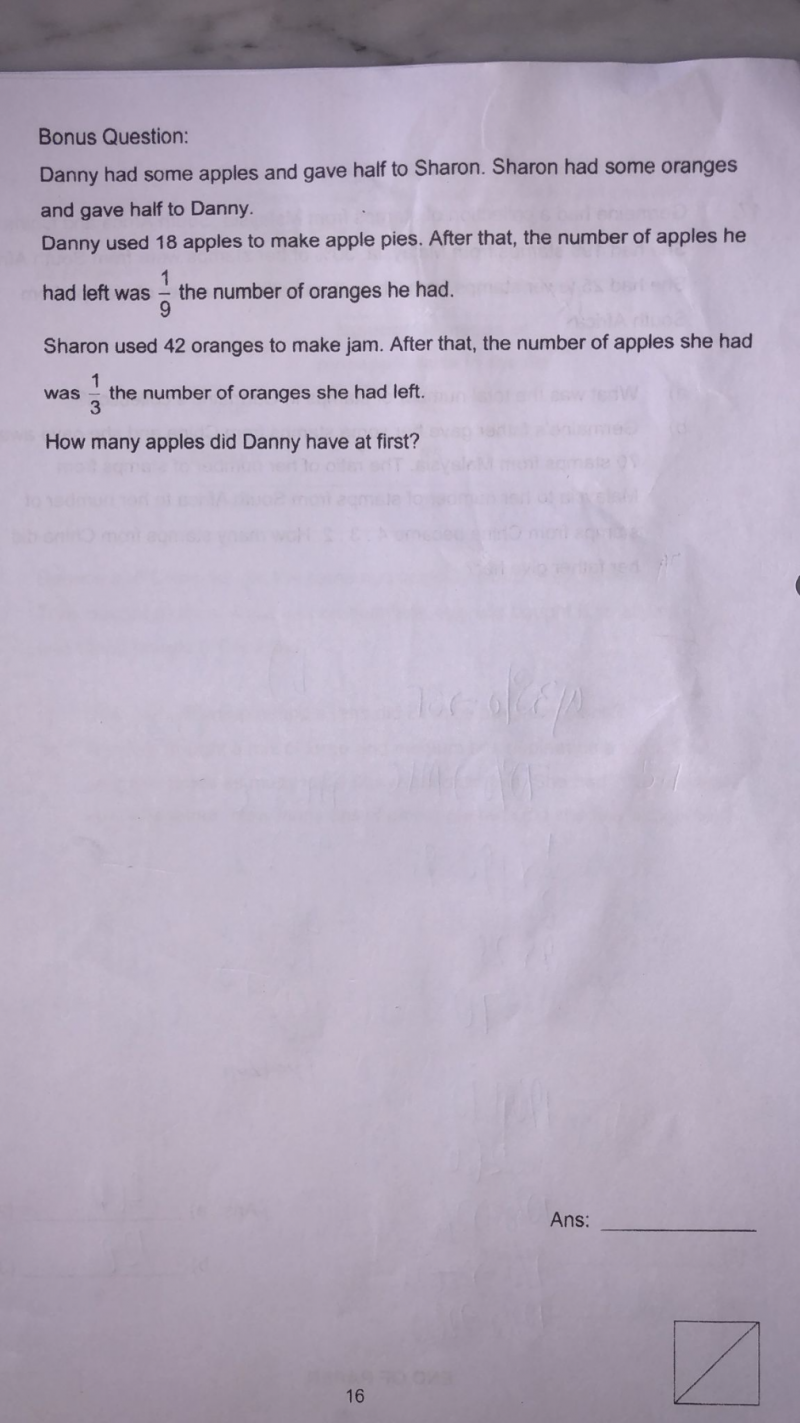# QuestionDanny’s Apples               [u]  where u = number of apples left after using 18 + after giving 1/2 to S

Danny’s Oranges                    [————9u————-]

Sharon’s Oranges           [—3p—]         where 3p = number of oranges left after using 42 + after giving 1/2 to D

Sharon’s Apples                      [p]

18 + u = p                (Danny gave half his apples to Sharon)

42 + 3p = 9u           (Sharon gave half her oranges to Danny)

42 + 3(18 + u) = 9u

42 + 54 + 3u = 9u

6u = 96 => u = 16

Hence number of apples Danny has at at first = 2 (18+16) = 68